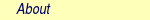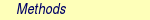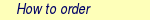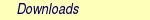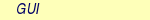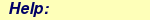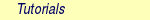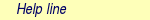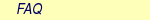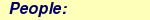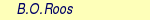MOLCAS manual:Next: 8.11 embq Up: 8. Programs Previous: 8.9 cpf

Subsections

# 8.10 dynamix

The DYNAMIX program performs molecular dynamics (MD) simulations in MOLCAS. Here the nuclei are moved according to the classical Newton's equations which are solved numerically using the velocity Verlet algorithm. The algorithm requires coordinates, velocities and forces as input. DYNAMIX can be used with any electronic structure method in MOLCAS. Also environmental effects can be taken into account in the MD simulation: the solvent can be considered implicitly using the reaction field keyword in GATEWAY or explicitly in hybrid QM/MM calculation which requires the ESPF program.

When multiple electronic states are involved in a MD simulation, a trajectory surface hopping (TSH) algorithm allows non-adiabatic transitions between different states. This TSH algorithm evaluates the change of the wavefunction along the trajectory and induces a hop if certain criteria a met (for further details read the RASSI section). In the current implementation the surface hopping algorithm can be used only with state averaged CASSCF wavefunction. However, an extension for CASPT2 and other methods are in preparation.

The Tully algorithm is available in a separate module Surfacehop.

## 8.10.1 Dependencies

The coordinates and the forces are required by the DYNAMIX program. DYNAMIX reads the initial coordinates from the RUNFILE and updates them in each iteration. In addition DYNAMIX depends on the ALASKA program, since it generates forces.

## 8.10.2 Files

### 8.10.2.1 Input files

 File Contents velocity.xyz Contains the initial velocities of the MD simulation.

### 8.10.2.2 Output files

 File Contents RUNFILE Trajectory information such as current time, velocities, etc. are stored in this file. md.xyz The coordinates for each step of the MD trajectory are saved here. md.energies The potential, kinetic and total energies are written to this file. In case of multiple electronic states, the energies of all roots are saved.

## 8.10.3 Input

This section describes the input syntax of DYNAMIX in the MOLCAS program package. In general a MD simulation requires a FOREACH loop which contains several programs to compute the energy and ALASKA for subsequent gradient computation. The input of the DYNAMIX begins with the program name, and is followed by the only compulsory keyword VELV which specifies the velocity Verlet algorithm:

&DYNAMIX
VELV

 Keyword Meaning VELVerlet This keyword specifies the velocity Verlet algorithm  to solve Newton's equations of motion. It's the only compulsory keyword in the program. DTime Defines thet which is the time step in the MD simulation and which is used for the integration of Newton's equations of motion. The program expects the time to be given in floating point format and in atomic unit of time (1 a.u. of time = 2.42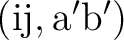10-17 s). (Default = 10). VELOcities Specifies how the initial velocities are generated. This keyword is followed by an integer on the next line. The internal unit of the velocities is [Bohr(a.u. of time)-1]. 0 - Zero velocities. (Default) 1 - The velocities are read from the file $Project.velocity.xyz in$WorkDir. This file contains velocities in the xyz format given in the same order as the atoms in coordinate file. The unit of the velocities is [Bohr(a.u. of time)-1]. 2 - This option allows to read in mass-weighted velocities from the file $Project.velocity.xyz in [Bohr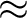(a.u. of time)-1]. 3 - This option takes random velocities from a Maxwell-Boltzmann distribution, at a given temperature, assuming that every component of the velocity can be considered as an independent gaussian random variable. THERmostat Regulates the control of the temperature by scaling the velocities. The option is an integer given on the next line. 0 - No velocity scaling. (Default) 1 - The velocities are scaled in order to keep the total energy constant. 2 - The velocities are scaled according to the Nosé-Hoover chain of thermostats algorithm, used to perform molecular symulation at constant temperature, resulting in statistics belonging to the canonical ensemble (NVT). TEMPerature Defines the numerical value of the temperature, which is used together with the Nosé-Hoover chain of thermostats to perform molecular dynamics at constant temperature. (Default = 298.15K) HOP Enables the trajectory surface hopping algorithm if the integer given in the next line is bigger than 0. The integer also specifies how many non-adiabatic transitions are allowed between electronic states. RESTART This keyword allows to restart the trajectory at a given time. The time is given on the next line in atomic units. H5RESTART This keyword allows to restart a trajectory calculation from an HDF5 file. The name of the restart file is given on the next line. ### 8.10.3.2 Input examples The following example shows the input for an excited state CASSCF molecular dynamics simulation of a methaniminium cation using the DYNAMIX program. The FOREACH loop allows 1000 steps with 10 a.u. of time step size which leads to a total duration of 242 fs. In the RASSCF program the second root is selected for gradient calculation using the keyword MDRLXR. This input assumes that the a JOBIPH file with orbitals is already given. In each iteration the JOBIPH is updated to achieve a fast convergence of the CASSCF wavefunction. A Nosé-Hoover chain of thermostats, enabled with THERmo= 2, is used to reproduce dynamics at constant temperature, where the initial velocities are taken from a Maxwell-Boltzmann distribution at 300 K. &GATEWAY COORD 6 Angstrom C 0.00031448 0.00000000 0.04334060 N 0.00062994 0.00000000 1.32317716 H 0.92882820 0.00000000 -0.49115611 H -0.92846597 0.00000000 -0.49069213 H -0.85725321 0.00000000 1.86103989 H 0.85877656 0.00000000 1.86062860 BASIS= 3-21G GROUP= nosym >> FOREACH ITER in (1 .. 1000) &SEWARD >> IF ($ITER  =  1  )

&RASSCF
LUMORB
FileOrb=  $Project.GssOrb Symmetry= 1 Spin= 1 nActEl= 2 0 0 Inactive= 7 RAS2= 2 CIroot= 3 3 1 >> COPY$Project.JobIph  $Project.JobOld >> ENDIF &RASSCF JOBIPH; CIRESTART Symmetry= 1 Spin= 1 nActEl= 2 0 0 Inactive= 7 RAS2= 2 CIroot= 3 3 1 MDRLXR= 2 >> COPY$Project.JobIph  \$Project.JobOld

&DYNAMIX
VELVer
DT=  10.0
VELO=  3
THER=  2
TEMP=300
HOP=  1

>>  END  DO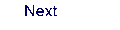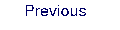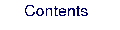Next: 8.11 embq Up: 8. Programs Previous: 8.9 cpf